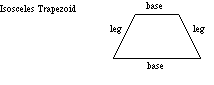index: click on a letter A B C D E F G H I J K L M N O P Q R S T U V W X Y Z A to Z index index: subject areas numbers & symbols sets, logic, proofs geometry algebra trigonometry advanced algebra & pre-calculus calculus advanced topics probability & statistics real world applications multimedia entrieswww.mathwords.com about mathwords website feedback

 Isosceles Trapezoid A trapezoid with base angles that are the same. Consequently, the legs will be congruent to each other as well.Note: This is US usage. In the UK this figure would be called an isosceles trapezium. See also